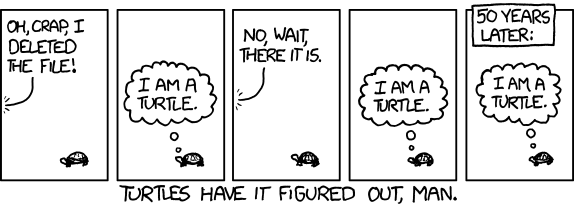# Mirror Image

## XKCD turtlesI’m Achilles!
I’m a turtle
I’m Spartacus!
I’m a turtle
I think therefore I am!
I’m a turtle
I’m ClearCase!
I’m a turtle
I am the alpha and the omega!
I’m a turtle

via xkcd

7, May, 2011 Posted by | sci | , | 2 Comments

## Robust estimators III: Into the deep

Cauchy estimator have some nice properties (Gonzales et al “Statistically-Efficient Filtering in Impulsive Environments: Weighted Myriad Filter” 2002):
By tuning$\gamma$ in$\psi(x) = \frac{x}{\gamma^2+ x^2}$
it can approximate either least squares (big$\gamma$), or mode – maximum of histogram – of sample set (small$\gamma$). For small$\gamma$ estimator behave the same way as power law distribution estimator with small$\alpha$.
Another property is that for several measurements with different scales$\gamma_i$ estimator of their sum will be simple$\psi(x) = \frac{x}{(\sum \gamma_i)^2+ x^2}$
which is convenient for estimation of random walks

I heard convulsion in the sky,
And flight of angel hosts on high,
And monsters moving in the deep

Those verses from The Prophet by A.Pushkin could be seen as metaphor of profound mathematical insight, encompassing bifurcations, higher dimensional algebra and murky depths of statistics.
I now intend to dive deeper into of statistics – toward “data depth”. Data depth is a generalization of median concept to multidimensional data. Remind you that median can be seen either as order parameter – value dividing the higher half of measurements from lower, or geometrically, as the minimum of$L_1$ norm. Second approach lead to geometric median, about which I already talked about.
First approach to generalizations of median is to try to apply order statistics to multidimensional vectors.The idea is to make some kind of partial order for n-dimensional points – “depth” of points, and to choose as the analog of median the point of maximum depth.
Basically all data depth concepts define “depth” as some characterization of how deep points are reside inside the point cloud.
Historically first and easiest to understand was convex hull approach – make convex hull of data set, assign points in the hull depth 1, remove it, get convex hull of points remained inside, assign new hull depth 2, remove etc.; repeat until there is no point inside last convex hull.
Later Tukey introduce similar “halfspace depth” concept – for each point X find the minimum number of points which could be cut from the dataset by plane through the point X. That number count as depth(see the nice overview of those and other geometrical definition of depth at Greg Aloupis page)
In 2002 Mizera introduced “global depth”, which is less geometric and more statistical. It start with assumption of some loss function (“criterial function” in Mizera definition)$F(x_i, \theta)$ of measurement set$x_i$. This function could be(but not necessary) cumulative probability distribution. Now for two parameters$\theta_1$ and$\theta_2$,$\theta_1$ is more fit with respect$A \subset N$ if for all$i \in A$$F(x_i, \theta_1) > F(x_i, \theta_2)$.$\hat{\theta}$ is weakly optimal with respect to$A$ if there is nor better fit parameter with respect to$A$. At last global depth of$\theta$ is the minimum possible size of$A$ such that$\theta$ is not weakly optimal with respect to$N \setminus A$ – reminder of measurements. In other words global depth is minimum number of measurements which should be removed for$\theta$ stop being weakly optimal. Global depth is not easy to calculate or visualize, so Mizera introduce more simple concept – tangent depth.
Tangent depth defined as$min_{\parallel u\parallel=1}\mid \{ i: u^T \bigtriangledown_{\theta} F(x_i) \geq 0 \}\mid$. What does it mean? Tangent depth is minimum number of “bad” points – such points that for specific direction loss function for themis growing.
Those definitions of “data depth” allow for another type of estimator, based not on likelihood, but on order statisticsmaximum depth estimators. The advantage of those estimators is robustness(breakdown point ~25%-33%) and disadvantage – low precision (high bias). So I wouldn’t use them for precise estimation, but for sanity check or initial approximation. In some cases they could be computationally more cheap than M-estimators. As useful side effect they also give some insight into structure of dataset(it seems originally maximum depth estimators was seen as data visualization tool). Depth could be good criterion for outliers rejection.
Disclaimer: while I had very positive experience with Cauchy estimator, data depth is a new thing for me.I have yet to see how useful it could be for computer vision related problems.

2, May, 2011 Posted by | computer vision, sci | , , , | Comments Off on Robust estimators III: Into the deep